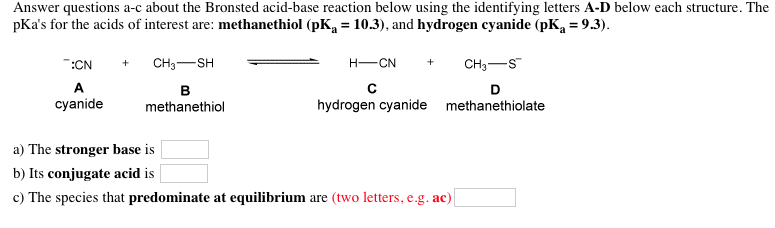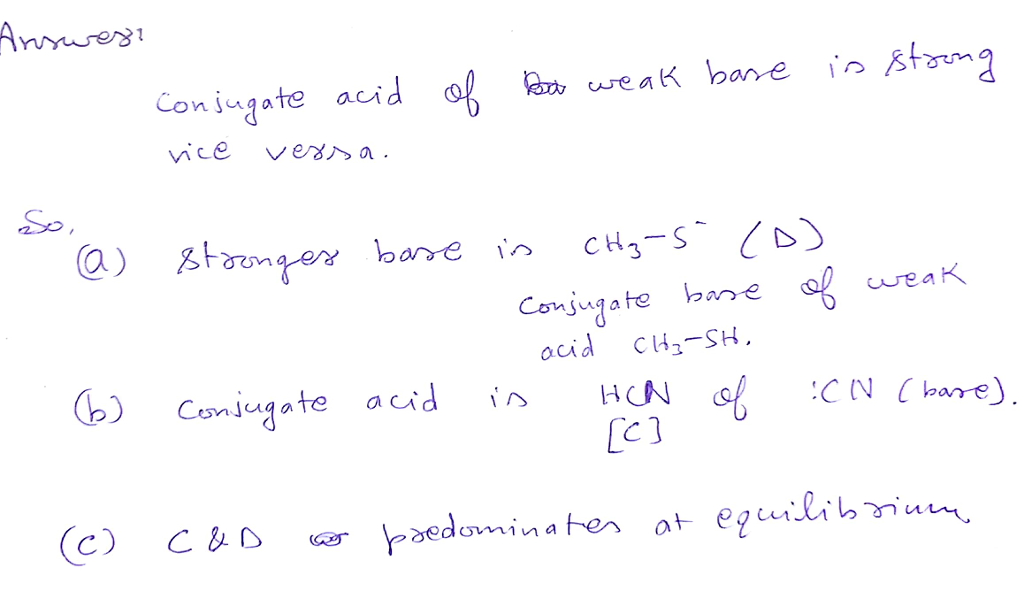# Question & Answer: Answer questions a-c about the Bronsted acid-base reaction below using the identifying let…..Answer questions a-c about the Bronsted acid-base reaction below using the identifying letters A-D below each structure. The pKa’s for the acids of interest are: methanethiol (pK_a = 10.3), and hydrogen cyanide (pK_a = 9.3). a) The stronger base is b) Its conjugate acid is c) The species that predominate at equilibrium are (two letters, e.g. ac)